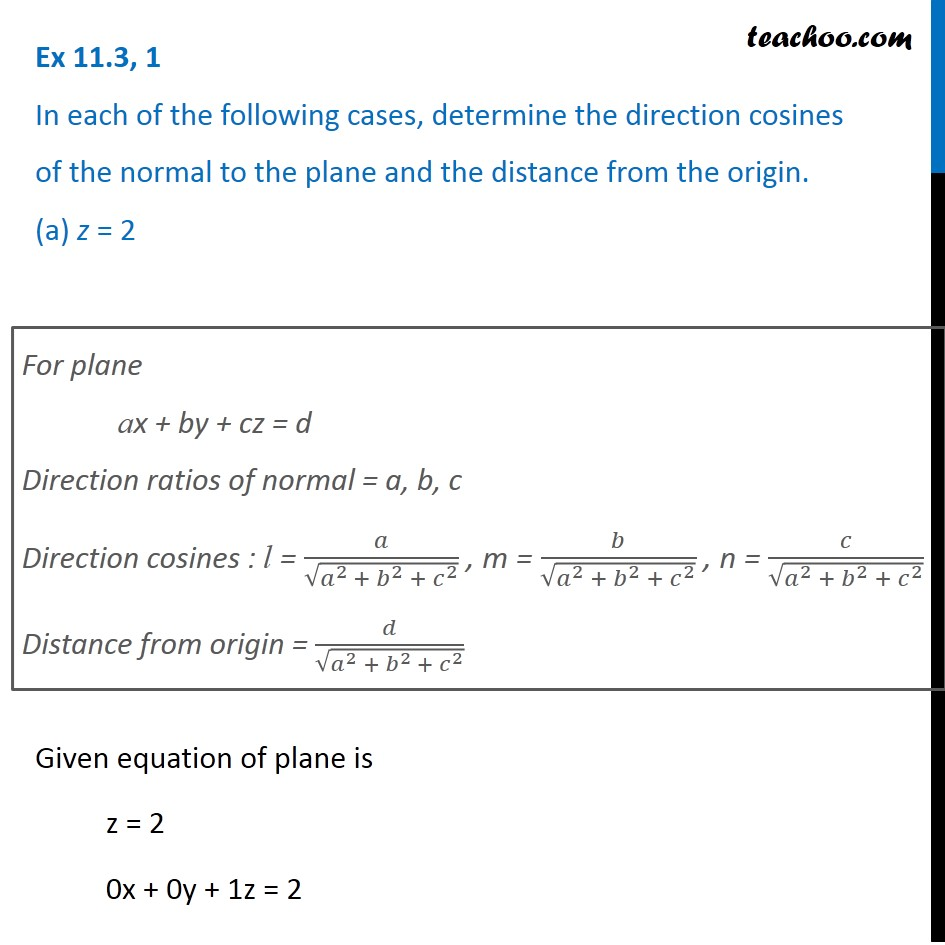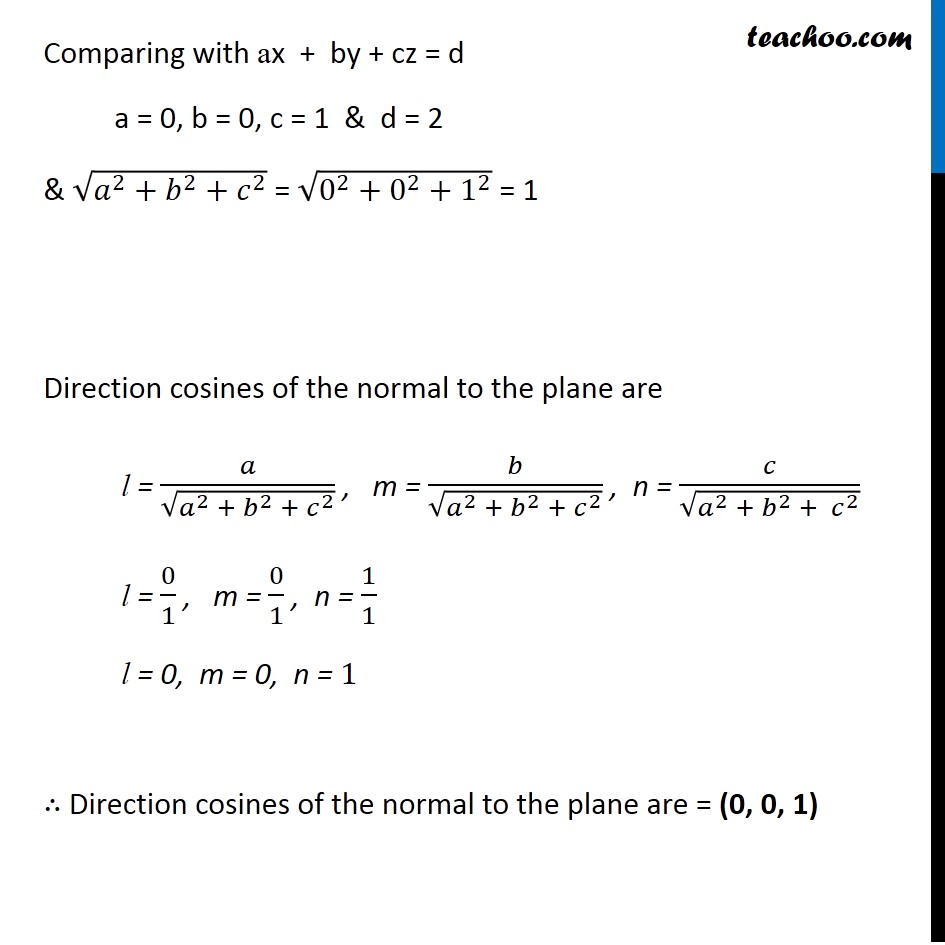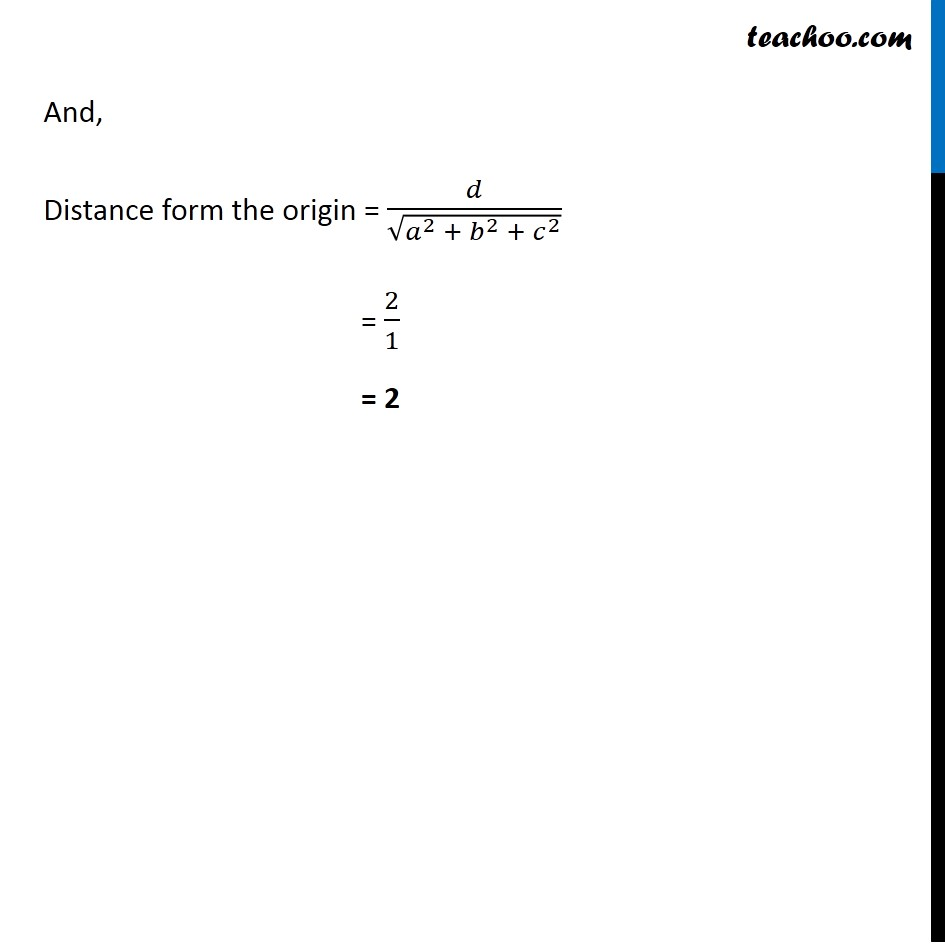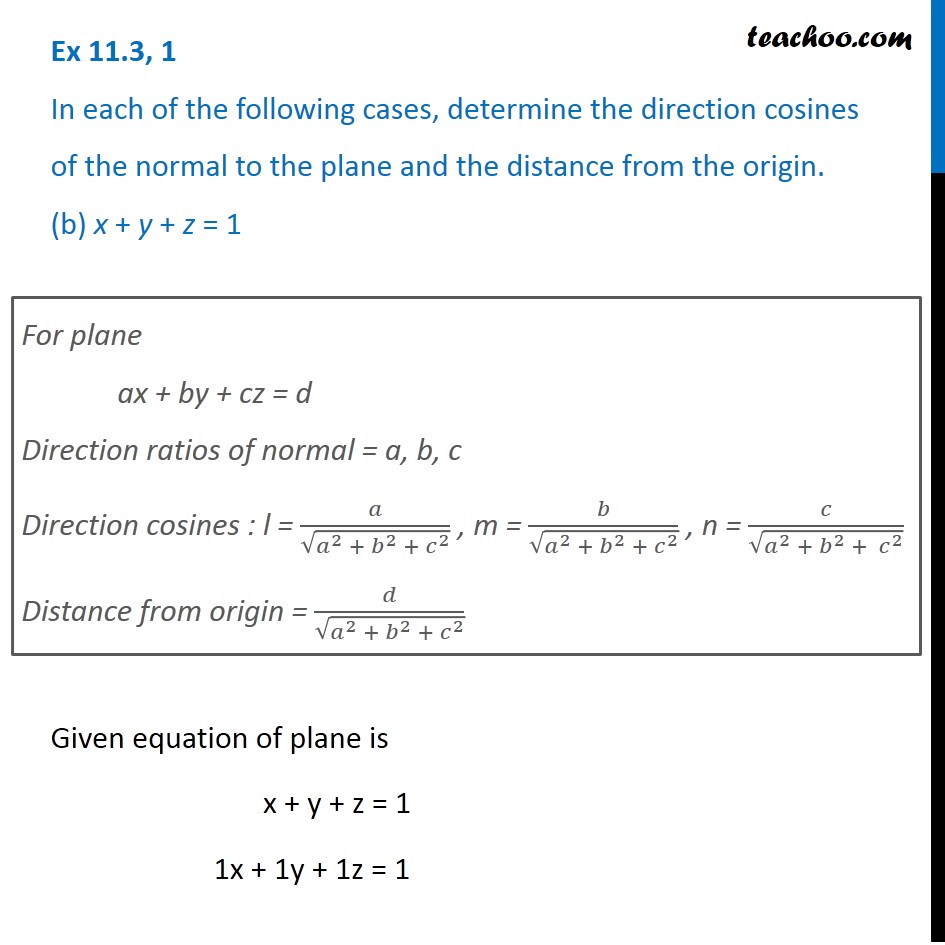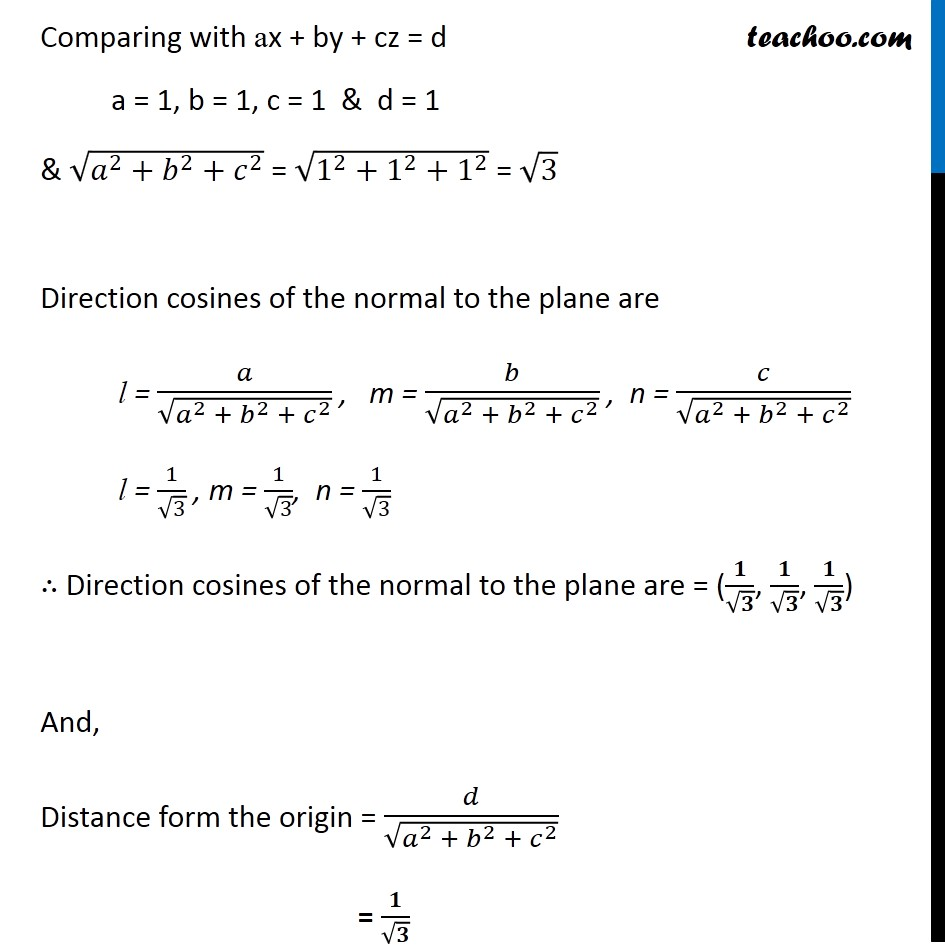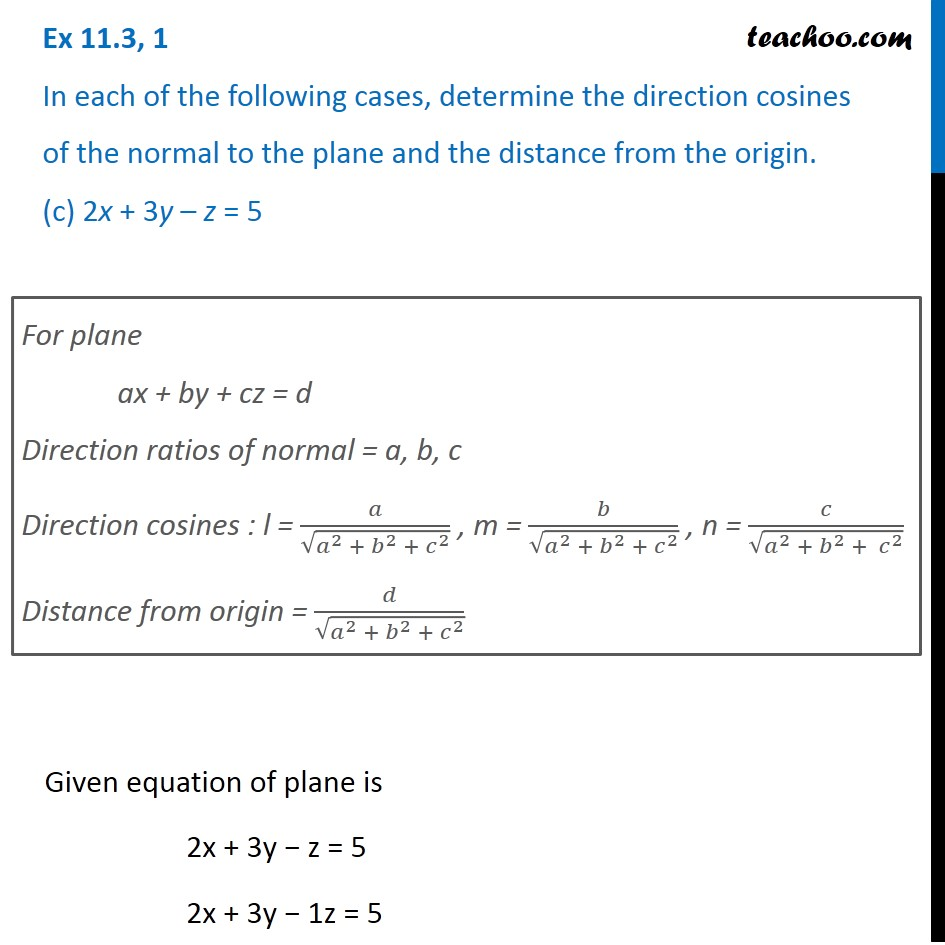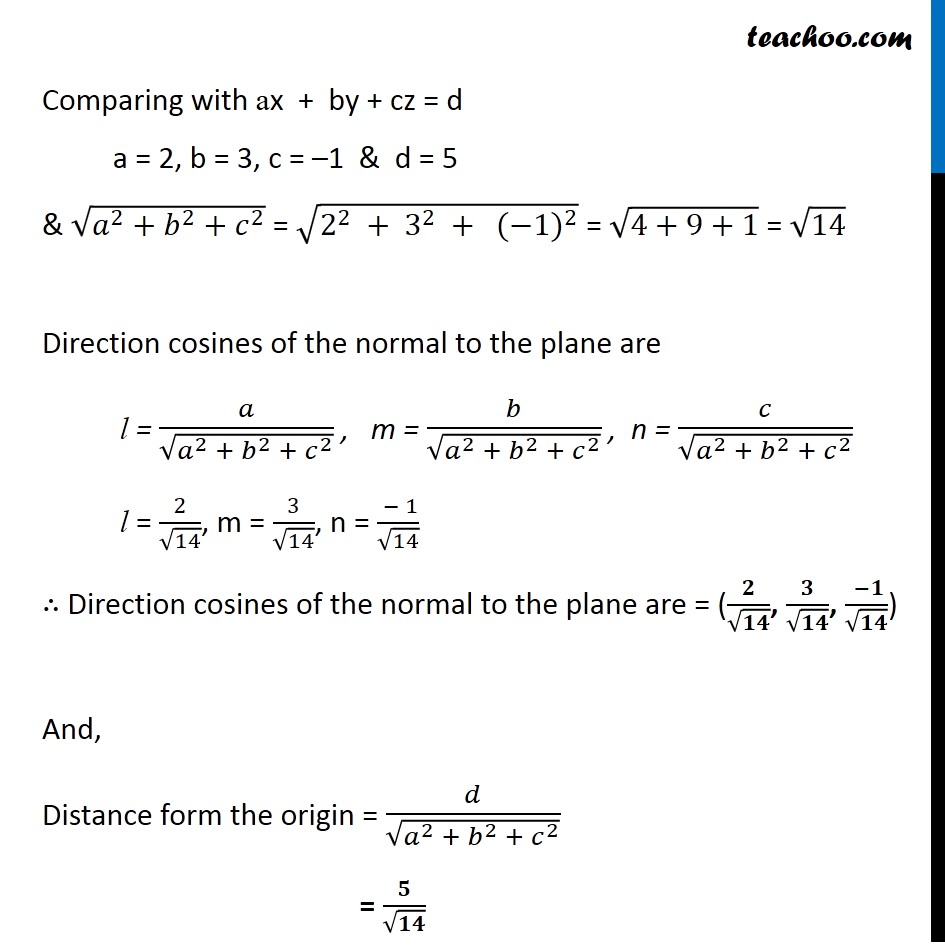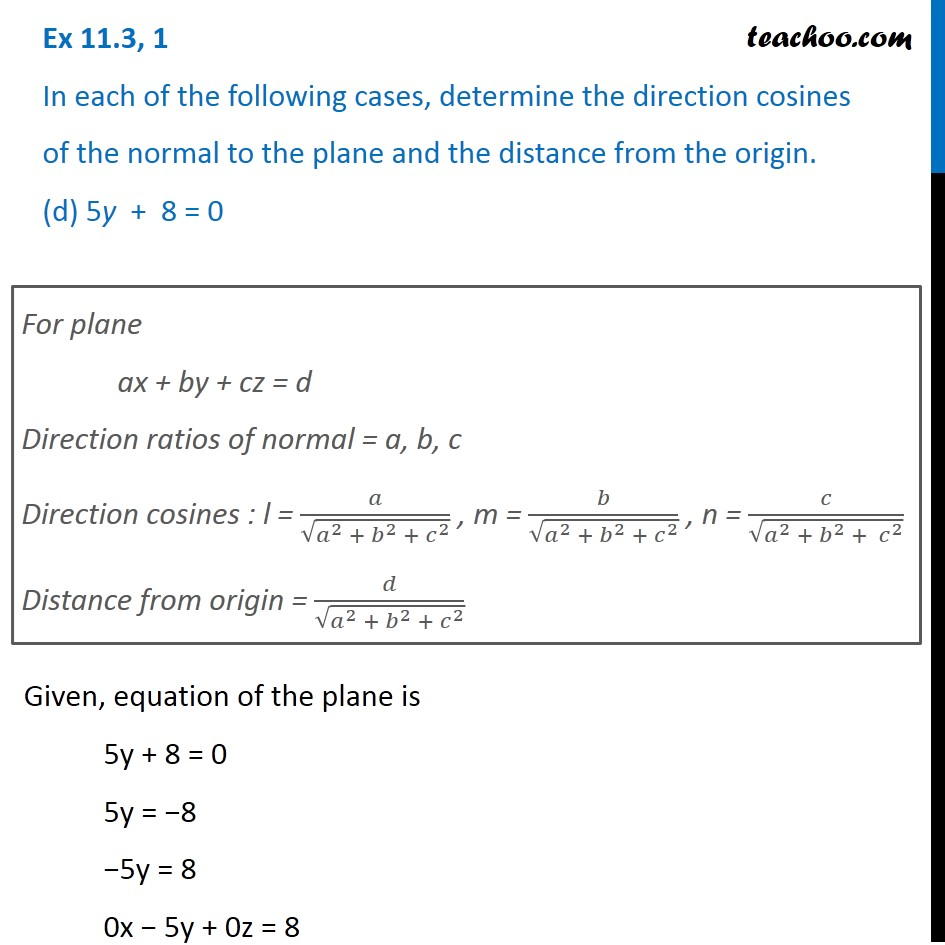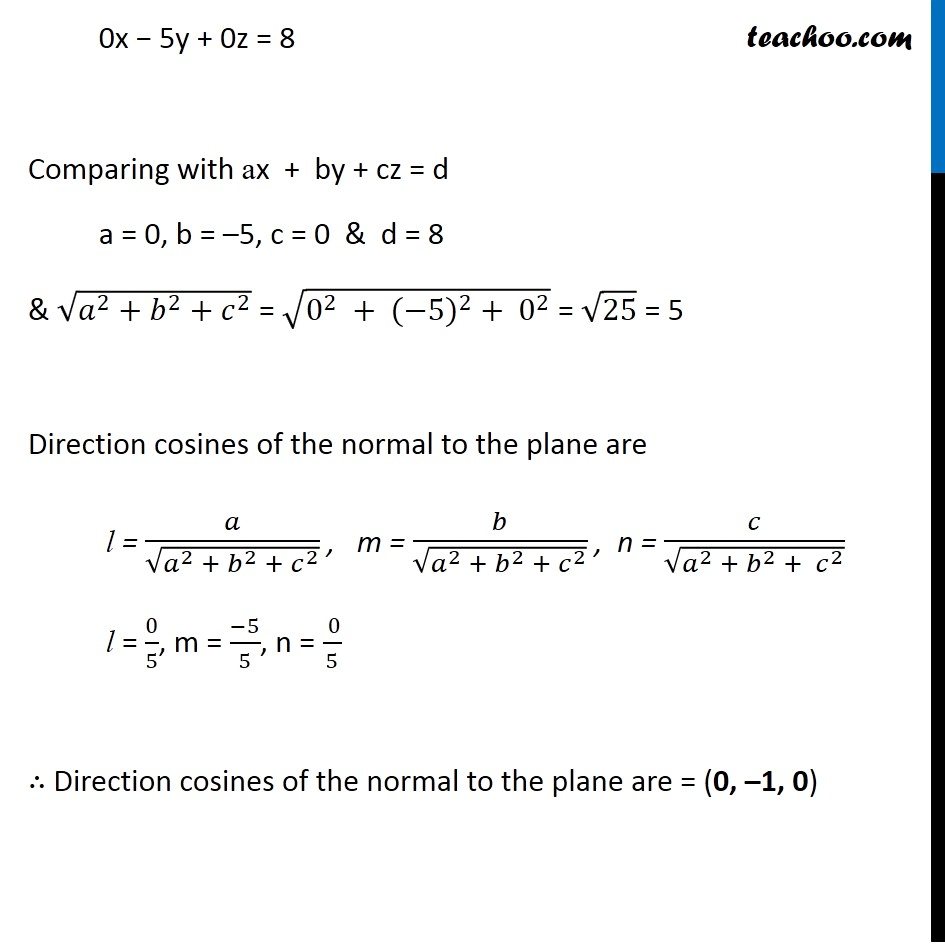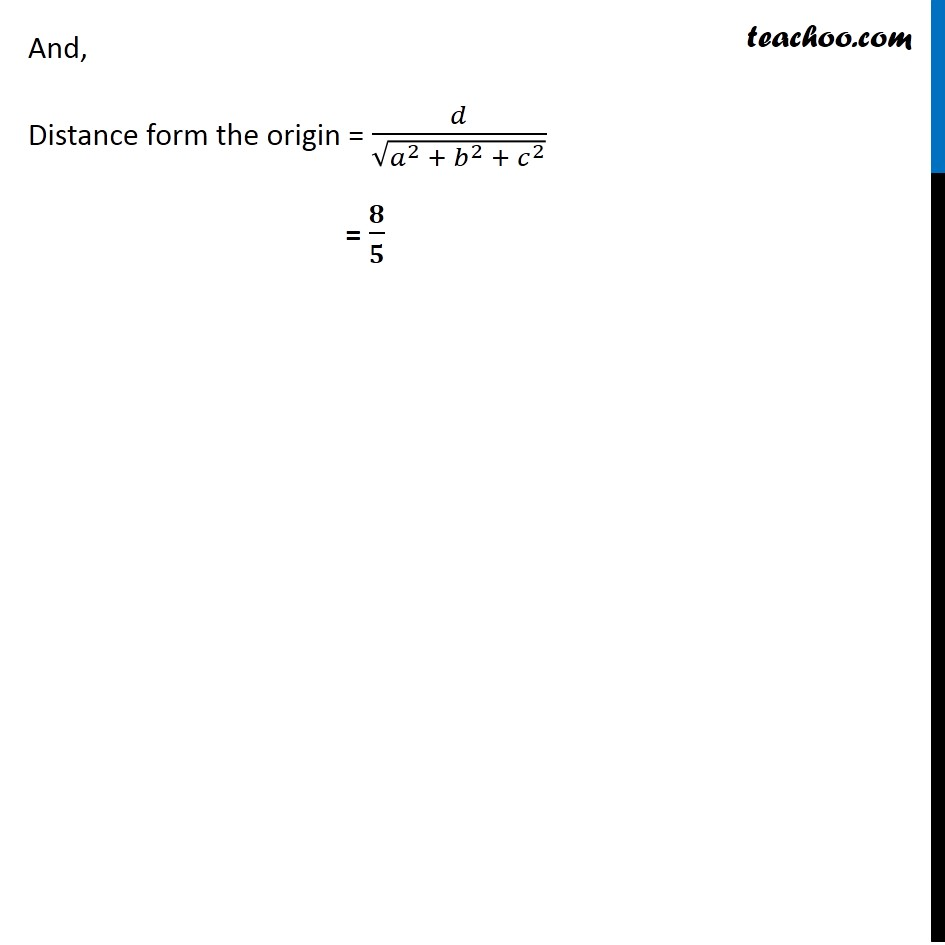Subscribe to our Youtube Channel - https://you.tube/teachoo

1. Chapter 11 Class 12 Three Dimensional Geometry
2. Serial order wise
3. Ex 11.3

Transcript

Ex 11.3, 1 In each of the following cases, determine the direction cosines of the normal to the plane and the distance from the origin. (a) z = 2 For plane ax + by + cz = d Direction ratios of normal = a, b, c Direction cosines : l = 𝑎/√(𝑎^(2 )+ 𝑏^2 + 𝑐^2 ) , m = 𝑏/√(𝑎^2 +〖 𝑏〗^2 + 𝑐^2 ) , n = 𝑐/√(𝑎^2 + 𝑏^2 + 𝑐^2 ) Distance from origin = 𝑑/√(𝑎^2 + 𝑏^(2 )+ 𝑐^2 ) Given equation of plane is z = 2 0x + 0y + 1z = 2 Comparing with ax + by + cz = d a = 0, b = 0, c = 1 & d = 2 & √(𝑎^2+𝑏^2+𝑐^2 ) = √(0^2+0^2+1^2 ) = 1 Direction cosines of the normal to the plane are l = 𝑎/√(𝑎^2 + 𝑏^2 + 𝑐^2 ) , m = 𝑏/√(𝑎^2 + 𝑏^2 + 𝑐^2 ) , n = 𝑐/√(𝑎^2 + 𝑏^2 + 𝑐^2 ) l = 0/1 , m = 0/1 , n = 1/1 l = 0, m = 0, n = 1 ∴ Direction cosines of the normal to the plane are = (0, 0, 1) And, Distance form the origin = 𝑑/√(𝑎^2 + 𝑏^2 + 𝑐^2 ) = 2/1 = 2 Ex 11.3, 1 In each of the following cases, determine the direction cosines of the normal to the plane and the distance from the origin. (b) x + y + z = 1 For plane ax + by + cz = d Direction ratios of normal = a, b, c Direction cosines : l = 𝑎/√(𝑎^2 + 𝑏^2 +〖 𝑐〗^2 ) , m = 𝑏/√(𝑎^2 + 𝑏^2 + 𝑐^2 ) , n = 𝑐/√(𝑎^2 + 𝑏^2 + 𝑐^2 ) Distance from origin = 𝑑/√(𝑎^2 + 𝑏^2 + 𝑐^2 ) Given equation of plane is x + y + z = 1 1x + 1y + 1z = 1 Comparing with ax + by + cz = d a = 1, b = 1, c = 1 & d = 1 & √(𝑎^2+𝑏^2+𝑐^2 ) = √(1^2+1^2+1^2 ) = √3 Direction cosines of the normal to the plane are l = 𝑎/√(𝑎^2 + 𝑏^2 + 𝑐^2 ) , m = 𝑏/√(𝑎^2 + 𝑏^2 + 𝑐^2 ) , n = 𝑐/√(𝑎^2 + 𝑏^2 + 𝑐^2 ) l = 1/√3 , m = 1/√3, n = 1/√3 ∴ Direction cosines of the normal to the plane are = (𝟏/√𝟑, 𝟏/√𝟑, 𝟏/√𝟑) And, Distance form the origin = 𝑑/√(𝑎^2 + 𝑏^2 + 𝑐^2 ) = 𝟏/√𝟑 Ex 11.3, 1 In each of the following cases, determine the direction cosines of the normal to the plane and the distance from the origin. (c) 2x + 3y – z = 5 For plane ax + by + cz = d Direction ratios of normal = a, b, c Direction cosines : l = 𝑎/√(𝑎^2 + 𝑏^2 +〖 𝑐〗^2 ) , m = 𝑏/√(𝑎^2 + 𝑏^2 + 𝑐^2 ) , n = 𝑐/√(𝑎^2 + 𝑏^2 + 𝑐^2 ) Distance from origin = 𝑑/√(𝑎^2 + 𝑏^2 + 𝑐^2 ) Given equation of plane is 2x + 3y − z = 5 2x + 3y − 1z = 5 Comparing with ax + by + cz = d a = 2, b = 3, c = –1 & d = 5 & √(𝑎^2+𝑏^2+𝑐^2 ) = √(2^2 + 3^2 + 〖(−1)〗^2 ) = √(4+9+1) = √14 Direction cosines of the normal to the plane are l = 𝑎/√(𝑎^2 + 𝑏^2 + 𝑐^2 ) , m = 𝑏/√(𝑎^2 + 𝑏^2 + 𝑐^2 ) , n = 𝑐/√(𝑎^2 + 𝑏^2 + 𝑐^2 ) l = 2/√14, m = 3/√14, n = ( − 1)/√14 ∴ Direction cosines of the normal to the plane are = (𝟐/√𝟏𝟒, 𝟑/√𝟏𝟒, ( −𝟏)/√𝟏𝟒) And, Distance form the origin = 𝑑/√(𝑎^2 + 𝑏^2 +〖 𝑐〗^2 ) = 𝟓/√𝟏𝟒 Ex 11.3, 1 In each of the following cases, determine the direction cosines of the normal to the plane and the distance from the origin. (d) 5y + 8 = 0 For plane ax + by + cz = d Direction ratios of normal = a, b, c Direction cosines : l = 𝑎/√(𝑎^2 + 𝑏^2 +〖 𝑐〗^2 ) , m = 𝑏/√(𝑎^2 + 𝑏^2 + 𝑐^2 ) , n = 𝑐/√(𝑎^2 + 𝑏^2 + 𝑐^2 ) Distance from origin = 𝑑/√(𝑎^2 + 𝑏^2 + 𝑐^2 ) Given, equation of the plane is 5y + 8 = 0 5y = −8 −5y = 8 0x − 5y + 0z = 8 0x − 5y + 0z = 8 Comparing with ax + by + cz = d a = 0, b = –5, c = 0 & d = 8 & √(𝑎^2+𝑏^2+𝑐^2 ) = √(0^2 + 〖(−5)〗^2 + 0^2 ) = √25 = 5 Direction cosines of the normal to the plane are l = 𝑎/√(𝑎^2 + 𝑏^2 + 𝑐^2 ) , m = 𝑏/√(𝑎^2 + 𝑏^2 + 𝑐^2 ) , n = 𝑐/√(𝑎^2 + 𝑏^2 + 𝑐^2 ) l = 0/5, m = (−5)/5, n = ( 0)/5 ∴ Direction cosines of the normal to the plane are = (0, –1, 0) And, Distance form the origin = 𝑑/√(𝑎^2 + 𝑏^2 + 𝑐^2 ) = 𝟖/𝟓

Ex 11.3